# On Ostrowski-Type Inequalities for Higher-Order Partial Derivatives

## Abstract

We establish some new Ostrowski-type integral inequalities involving higher-order partial derivatives. As applications, we get some interrelated results. Our results provide new estimates on inequalities of this type.

## 1. Introduction

The following inequality is well known in the literature as Ostrowski's integral inequality (see [1, page 468]).

Theorem 1.1.

Letbe a differentiable mapping on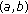whose derivativeis bounded on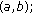that is,then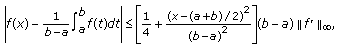(1.1)

for all.

Many generalizations, extensions and variations of this inequality have appeared in the literature; see  and the references given therein. In particular, in 2009, Wang and Zhao  established a new Ostrowski-type inequality for higher-order derivatives as follows (see  for definitions and notations):(1.2)

The main purpose of the present paper is to establish the following Ostrowski-type inequality involving higher-order partial derivatives (see next section for definitions and notations):(1.3)

where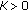is a constant,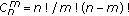,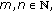andThis is a generalization of inequality (1.2).

Moreover, as applications, we get some interrelated results. Our results provide new estimates on such type of inequalities.

## 2. Main Results

Theorem 2.1.

Suppose that

(1)is continuous on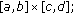(2)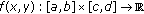is differentiable in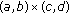up to order, with boundedth-order mixed partial derivatives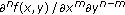(are natural numbers, and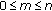), that is,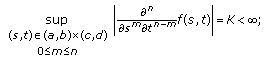(2.1)

(3)there exists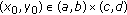such that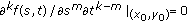,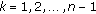;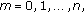then for any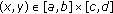,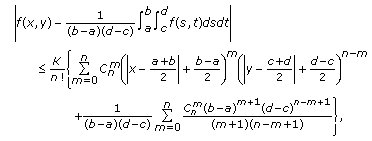(2.2)

whereProof.

From the hypotheses and using the-dimensional Taylor expansion ofat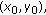we have, for some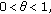(2.3)

Dividing both sides of (2.3) by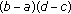, then integrating overfromtofirst, and then integrating the resulting inequality overfromto, we observe that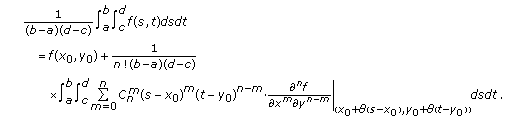(2.4)

Note that we have replaced the dummy variables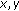by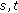, respectively. From (2.3) and (2.4), we have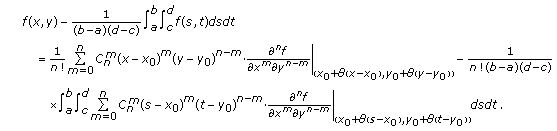(2.5)

Hence(2.6)

On the other hand, by applying the following two elementary inequalities :(2.7)

to the right-hand side of (2.6), we obtain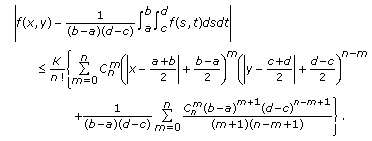(2.8)

This completes the proof.

Remark.

With suitable modifications, it is easy to see that (2.2) reduces to the following inequality in the 1-dimensional situation:(2.9)

where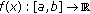is continuous on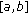, withth-order derivativebounded on, that is,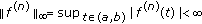, andObserve that this is a recent result of Wang and Zhao .

Theorem.

Suppose that

(1)is continuous on(2)is twice differentiable inwith bounded second-order partial derivatives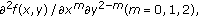that is,(2.10)

(3)there existssuch thatThen, one has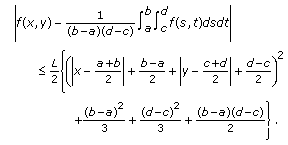(2.11)

Proof.

From the hypotheses and in view of the-dimensional Taylor expansion, it easily follows that for some,(2.12)

Hence,(2.13)

This proves Theorem 2.3.

Letand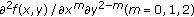change toand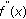, respectively, and with suitable modifications, Theorem 2.3 reduces to the following.

Theorem.

Suppose that

(1)is continuous on(2)is twice differentiable inwith bounded second -order derivative, that is,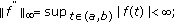(3)there exists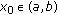such that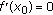(or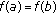),

then, one has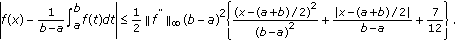(2.14)

This is another recent result of Wang and Zhao in .

## References

1. Mtrinović DS, Pečarić JE, Fink AM: Inequalities for Functions and Their Integrals and Dervatives. Kluwer Academic Publishers, Dordrecht, The Netherlands; 1994.

2. Pachpatte BG: On an inequality of Ostrowski type in three independent variables. Journal of Mathematical Analysis and Applications 2000, 249(2):583–591. 10.1006/jmaa.2000.6913

3. Pachpatte BG: On a new Ostrowski type inequality in two independent variables. Tamkang Journal of Mathematics 2001, 32(1):45–49.

4. Anastassiou GA: Multivariate Ostrowski type inequalities. Acta Mathematica Hungarica 1997, 76(4):267–278. 10.1023/A:1006529405430

5. Dragomir SS, Wang S: A new inequality of Ostrowski's type in norm and applications to some special means and to some numerical quadrature rules. Tamkang Journal of Mathematics 1997, 28(3):239–244.

6. Dragomir SS, Wang S: An inequality of Ostrowski-Grüss' type and its applications to the estimation of error bounds for some special means and for some numerical quadrature rules. Computers & Mathematics with Applications 1997, 33(11):15–20. 10.1016/S0898-1221(97)00084-9

7. Dragomir SS, Wang S: Applications of Ostrowski's inequality to the estimation of error bounds for some special means and for some numerical quadrature rules. Applied Mathematics Letters 1998, 11(1):105–109. 10.1016/S0893-9659(97)00142-0

8. Barnett NS, Dragomir SS: An Ostrowski type inequality for double integrals and applications for cubature formulae. RGMIA Research Report Collection 1998, 1: 13–23.

9. Baĭnov D, Simeonov P: Integral Inequalities and Applications, Mathematics and Its Applications. Volume 57. Kluwer Academic Publishers, Dordrecht, The Netherlands; 1992:xii+245.

10. Mitrinović DS, Pečarić JE, Fink AM: Inequalities Involving Functions and Their Integrals and Derivatives, Mathematics and Its Applications. Volume 53. Kluwer Academic Publishers, Dordrecht, The Netherlands; 1991:xvi+587.

11. Wang M, Zhao X: Ostrowski type inequalities for higher-order derivatives. Journal of Inequalities and Applications 2009, 2009:-8.

## Acknowledgments

The research is supported by the National Natural Sciences Foundation of China (10971205). It is partially supported by the Research Grants Council of the Hong Kong SAR, China (Project no. HKU7016/07P) and an HKU Seed Grant for Basic Research.

## Author information

Authors

### Corresponding author

Correspondence to Zhao Changjian.

## Rights and permissions

Reprints and Permissions

Changjian, Z., Cheung, WS. On Ostrowski-Type Inequalities for Higher-Order Partial Derivatives. J Inequal Appl 2010, 960672 (2010). https://doi.org/10.1155/2010/960672

• Accepted:

• Published:

• DOI: https://doi.org/10.1155/2010/960672

### Keywords

• Partial Derivative
• Natural Number
• Recent Result
• Taylor Expansion
• Differentiable Mapping